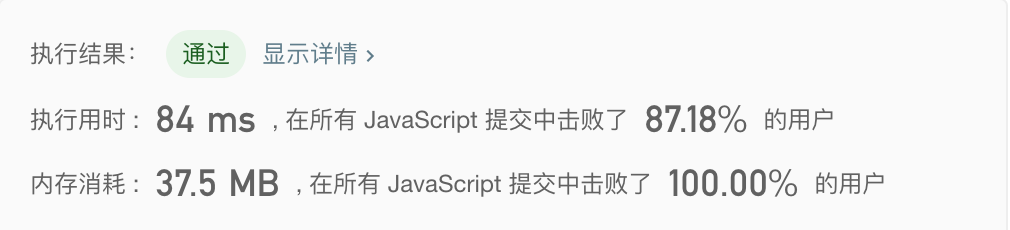K个一组翻转链表
•   更新：2020-05-16 21:10:05
•   首发：2020-05-16 21:04:29
•   源代码
•   1750

LeetCode 25. K 个一组翻转链表 https://leetcode-cn.com/problems/reverse-nodes-in-k-group/
LintCode 450. K组翻转链表 https://www.lintcode.com/problem/reverse-nodes-in-k-group/

K个一组翻转链表

``````/**
* @param {number} k
* @return {ListNode}
*/
export const reverseKGroup = function (head, k) {
let sum = 0 // 记录进行的结点个数
let start = head // 记录每次翻转的第一个元素
let res = head // 返回值：如果进行过翻转，则为第一次翻转的最后一个结点
const queue = [] // 使用队列，方便连接上一次翻转的链表，最大长度为2
if (++sum === k) { // 如果经过了k个结点，则翻转从start到head的一段结点
queue.push(start) // 计入队列
for (let i = 0; i < sum - 1; i++) { // 翻转结点
const tmp = start.next
start.next = next
next = start
start = tmp
}

start.next = next // 最后一个结点

if (queue.length === 1) { // 判断是否为第一次翻转
res = start
} else {
const la = queue.shift() // 连接上一次翻转的链表
}
sum = 0 // 重置计数
} else {
}
}

return res
}
``````上一篇 (Linux安装无线网卡驱动通用方法)